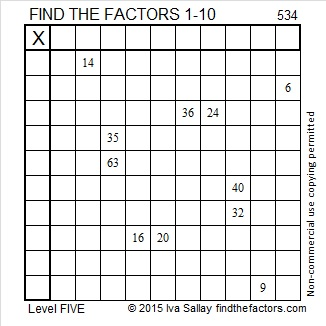# 534 and Level 5

534 is made of three consecutive digits so it can be evenly divided by three.

534 is the sum of consecutive primes: 127 + 131 + 137 + 139 = 534.

534 is the hypotenuse of the Pythagorean triple 234-480-534. Can you find the greatest common factor of those three numbers?Print the puzzles or type the solution on this excel file: 10 Factors 2015-06-22

—————————————————————————————————

• 534 is a composite number.
• Prime factorization: 534 = 2 x 3 x 89
• The exponents in the prime factorization are 1, 1, and 1. Adding one to each and multiplying we get (1 + 1)(1 + 1)(1 + 1) = 2 x 2 x 2 = 8. Therefore 534 has exactly 8 factors.
• Factors of 534: 1, 2, 3, 6, 89, 178, 267, 534
• Factor pairs: 534 = 1 x 534, 2 x 267, 3 x 178, or 6 x 89
• 534 has no square factors that allow its square root to be simplified. √534 ≈ 23.108440.—————————————————————————————————